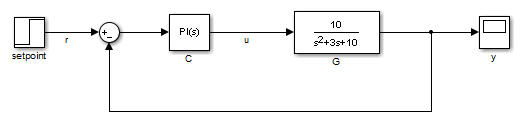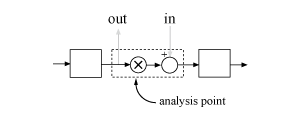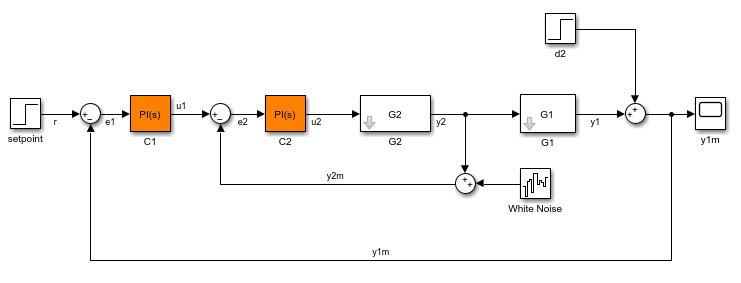Main Content

## Mark Signals of Interest for Batch Linearization

When batch linearizing a model using an `slLinearizer` interface, you can mark signals of interest using analysis points. You can then analyze the response of your system at any of these points using functions such as `getIOTransfer` and `getLoopTransfer`.

Alternatively, if you are batch linearizing your model using the:

For more information on selecting a batch linearization tool, see Choose Batch Linearization Methods.

### Analysis Points

Analysis points identify locations within a Simulink® model that are relevant for linear analysis. Each analysis point is associated with a signal that originates from the outport of a Simulink block. For example, in the following model, the reference signal `r` and the control signal `u` are analysis points that originate from the outputs of the setpoint and C blocks respectively.Each analysis point can serve one or more of the following purposes:

• Input — The software injects an additive input signal at an analysis point, for example, to model a disturbance at the plant input.

• Output — The software measures the signal value at a point, for example, to study the impact of a disturbance on the plant output.

• Loop Opening — The software interprets a break in the signal flow at a point, for example, to study the open-loop response at the plant input.

When you use an analysis point for more than one purpose, the software applies the purposes in this sequence: output measurement, then loop opening, then input.Using analysis points, you can extract open-loop and closed-loop responses from a Simulink model. You can also specify requirements for control system tuning using analysis points. For more information, see Mark Signals of Interest for Control System Analysis and Design.

### Specify Analysis Points

You can mark analysis points either explicitly in the Simulink model, or programmatically using the `addPoint` command for an `slLinearizer` interface.

#### Mark Analysis Points in Simulink Model

To specify analysis points directly in your Simulink model, first open the Linearization tab. To do so, in the Apps gallery, click Linearization Manager.

To specify an analysis point:

1. In the model, click the signal you want to define as an analysis point.

2. On the Linearization tab, in the Insert Analysis Points gallery, select the type of analysis point you want to define.

When you specify analysis points, the software adds annotations to your model indicating the linear analysis point type.

3. Repeat steps 1 and 2 for all signals you want to define as analysis points.

You can select any of the following closed-loop analysis point types, which are equivalent within an `slLinearizer` interface.

• Input Perturbation

• Output Measurement

• Sensitivity

• Complementary Sensitivity

If you want to introduce a permanent loop opening at a signal as well, select one of the following open-loop analysis point types:

• Open-Loop Input

• Open-Loop Output

• Loop Transfer

• Loop Break

When you define a signal as an open-loop point, analysis functions such as `getIOTransfer` always enforce a loop break at that signal during linearization. All open-loop analysis point types are equivalent within an `slLinearizer` interface. For more information on how the software treats loop openings during linearization, see How the Software Treats Loop Openings.

When you create an `slLinearizer` interface for a model, any analysis points defined in the model are automatically added to the interface. If you defined an analysis point using:

• A closed-loop type, the signal is added as an analysis point only.

• An open-loop type, the signal is added as both an analysis point and a permanent opening.

#### Mark Analysis Points Programmatically

To mark analysis points programmatically, use the `addPoint` command. For example, consider the `scdcascade` model.

```open_system('scdcascade') ```To mark analysis points, first create an `slLinearizer` interface.

```sllin = slLinearizer('scdcascade'); ```

To add a signal as an analysis point, use the `addPoint` command, specifying the source block and port number for the signal.

```addPoint(sllin,'scdcascade/C1',1); ```

If the source block has a single output port, you can omit the port number.

```addPoint(sllin,'scdcascade/G2'); ```

For convenience, you can also mark analysis points using the:

• Name of the signal.

```addPoint(sllin,'y2'); ```
• Combined source block path and port number.

```addPoint(sllin,'scdcascade/C1/1') ```
• End of the full source block path when unambiguous.

```addPoint(sllin,'G1/1') ```

You can also add permanent openings to an `slLinearizer` interface using the `addOpening` command, and specifying signals in the same way as for `addPoint`. For more information on how the software treats loop openings during linearization, see How the Software Treats Loop Openings.

```addOpening(sllin,'y1m'); ```

You can also define analysis points by creating linearization I/O objects using the `linio` command.

```io(1) = linio('scdcascade/C1',1,'input'); io(2) = linio('scdcascade/G1',1,'output'); addPoint(sllin,io); ```

As when you define analysis points directly in your model, if you specify a linearization I/O object with:

• A closed-loop type, the signal is added as an analysis point only.

• An open-loop type, the signal is added as both an analysis point and a permanent opening.

### Refer to Analysis Points

Once you have marked analysis points in an `slLinearizer` interface, you can analyze the response at any of these points using the following analysis functions:

To view the available analysis points in an `slLinearizer` interface, use the `getPoints` command.

```getPoints(sllin) ```
```ans = 3x1 cell array {'scdcascade/C1/1[u1]'} {'scdcascade/G2/1[y2]'} {'scdcascade/G1/1[y1]'} ```

To use an analysis point with an analysis function, you can specify an unambiguous abbreviation of the analysis point name returned by `getPoints`. For example, compute the transfer function from `u1` to `y1`, and find the sensitivity to a disturbance at the output of block G2.

```ioSys = getIOTransfer(sllin,'u1','y1'); sensG2 = getSensitivity(sllin,'G2'); ```

## SupportGet trial now